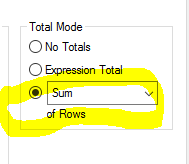# QlikView Layout & Visualizations

Discussion Board for collaboration on QlikView Layout & Visualizations.

Announcements
Modernize Your QlikView Deployment webinar, Nov. 3rd. REGISTER
cancel
Showing results for
Did you mean:
HighlightedCreator III

## Expression Condition

I have problem with this expression:

=if(Sum(CONSUMO_TOTALE_KWH)>=2000000,Sum(CONSUMO_TOTALE_KWH),0)

i need to put another condition like this:

Sum(CONSUMO_TOTALE_KWH)>=20000000

but if i put it in the IF expression, the result is: 0

How can i do  an expression to show me the SUM of my field CONSUMO_TOTALE_KWH where it need >= 2000000 and <= 20000000

1 Solution

Accepted Solutions
HighlightedMVP

Try this

Sum({<POD = {"=Sum(CONSUMO_TOTALE_KWH) >= 2000000 and Sum(CONSUMO_TOTALE_KWH) <= 20000000"}>} CONSUMO_TOTALE_KWH)

7 Replies
HighlightedMVP

May be like:

=if(

Sum(CONSUMO_TOTALE_KWH)>=2000000

AND

Sum(CONSUMO_TOTALE_KWH)<=20000000 ,

Sum(CONSUMO_TOTALE_KWH),

0)

HighlightedCreator III

=if(Sum(CONSUMO_TOTALE_KWH)>=2000000 and Sum(CONSUMO_TOTALE_KWH) <= 20000000,Sum(CONSUMO_TOTALE_KWH),0)

HighlightedCreator III

Hi, thanks for reply, but i have already did that, and it show me "0"

if i remove the second condition Sum(CONSUMO_TOTALE_KWH)<=20000000

it show me the value

why?HighlightedMVP

Try this

Sum({<POD = {"=Sum(CONSUMO_TOTALE_KWH) >= 2000000 and Sum(CONSUMO_TOTALE_KWH) <= 20000000"}>} CONSUMO_TOTALE_KWH)

HighlightedCreator III

in the expression tab you can use sum of rows.HighlightedCreator III

Great, this is OK!!!! Thanks, you!!

Can you explaint to me why the i set :

if(Sum(CONSUMO_TOTALE_KWH)>=2000000 and Sum(CONSUMO_TOTALE_KWH) <= 20000000,Sum(CONSUMO_TOTALE_KWH),0)

not work right,  and this you write is work ok? Thanks you Sunny

HighlightedMVP

This has to do with the fact that the total row also checks for the if statement... but at the total row the sum(CONSUMO_TOTALE_KWH) is most likely greater than 20000000 which is why it shows 0. In order to do sum of rows... you can try this

http://help.qlik.com/en-US/qlikview/November2017/Subsystems/Client/Content/ChartFunctions/NestedAggr...